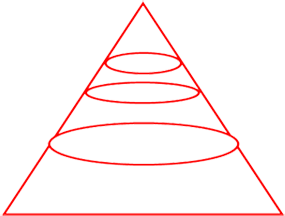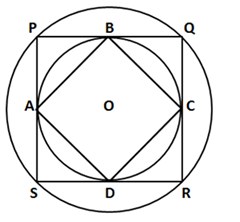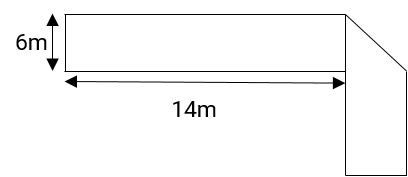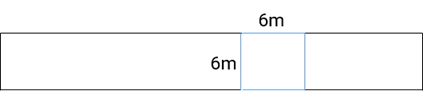#### Mensuration

Mensuration in Geometry is a fairly intuitive subject. Structural and spatial understanding of the forms, other than knowing simple formulae, makes one nail Mensuration questions in the test. Mensuration involves forms in 2D and 3D, including (but not limited to) circles, squares, cuboids, cones, cylinders, and more. If you have the formula in place, learn the measurement formulas such as those for cube, cuboid, cylinder, sphere, etc. for entrance exams as many problems can be addressed using the same. Due to the inevitability of strongly embedded geometric principles, various competitive exams such as CAT, XAT, SSC, BANK PO, etc. include an integral part of the course structure.

Don’t waste your time trying to answer it right away if you get stuck with a measurement question. Instead, when you are done with the rest of the manageable questions, get on with the others and come back to the topic.

CAT :
 Year No of Questions Good attempt Difficulty 2019 Slot 1 3 2 Moderate Slot 2 3 1 Difficult 2018 Slot 1 4 4 Easy Slot 2 5 3 or 4 Moderate

XAT :

 Year No of Questions Good attempt Difficulty 2020 4 2 Moderate 2019 1 1 Moderate

SNAP :

 Year No of Questions Good attempt Difficulty 2019 3 2 Easy-Moderate 2018 4 2 Moderate

### List of concepts in Mensuration

Mensuration Important Concepts

Impact of the cavity on a solid figure’s surface area

• Length of longest rod in cube shape room
• Impact of the solids melting to create new solids
• Slant surface and Entire Pyramid surface
• Dimensions of Pyramid etc.

Formula’s to learn, study and remember for are Curved Surface Area, Gross Surface Area, and Volume for solid figures like:

• Cube
• Cuboid
• Cylinder
• Cone
• Sphere
• Frustum
• Polygon
• Prism
• Pyramid etc.

### Solved Questions for Mensuration

Q1: The length, breadth, and height of a room are in the ratio 3:2:1. If the breadth and height are halved while the length is doubled, then the total area of the four walls of the room will (CAT 19 – Slot 1)

1. Remain the same
2. Dec. by 13.64%
3. Dec. by 15%
4. Dec. by 18.75%
5. Dec. by 30%

In the present case, let Length = l = 3x, Breadth = b = 2x, Height = h = x

Then, Area of four walls = 2 (l + b) h = 2(3x + 2x) x = 10×2.

Now as Length gets doubled = 6x, Breadth halved = x, Height halved = x/2.

New area of four walls = 2 (6x + x) x/2 = 7×2.

Thus there is a decrease of 30%. Hence, the fifth option is the answer.

Q2: In the following figure the smallest cone has a base radius of 2 cm and a height of 1 cm. The heights of successive cones from the top form a G.P. with 1 as the first term and 2 as the common ratio. What shall be the ratio of the volumes of the smallest cone, to the two following frustums? (CAT 12 – Slot 1)1. 1: 7: 56
2. 1: 8: 64
3. 3: 9: 18
4. None of these

The heights of successive cones are given to us as 1, 2, and 4.

The volumes will be equal to the cubes of the height (because volume = radius2x height) as the base radii will rise in the same proportion as the heights.

So, the volume ratio would be 13: 23: 43 = 1: 8: 64.

The question, however, is about the smallest cone of the frustum.

The smallest cone currently has a volume of 1, the smaller frustum has a volume of 7 (i.e. 8-1), and the larger frustum has a volume of 56 (i.e. 64 – 8). Hence the answer will be the first option.

Q3: The figure below shows two concentric circles with center O. PQRS is a square inscribed in the outer circle. It also circumscribes the inner circle, touching it at points B, C, D, and A. What is the ratio of the perimeter of the outer circle to that of polygon ABCD? (CAT 16 – Slot 2)1. π/4
2. 3π/2
3. π/2
4. π

Let the inner circle radius be r. On its circumference, A, B, C, and D are points where the sides of the square PQRS touch, meaning that the sides are tangent to the circle.

At the point of touch, the radius and the tangent are perpendiculars. Therefore, if we connect the OB and OC radius, then OB is perpendicular to PQ, OC is perpendicular to QR, OB = OC = r, then OBQC is a side square r.

The OQ is the diagonal = √2r = R = outer circle radius. BC is also the diagonal of OQ = R

ABCD is a square of side R and the radius of the outer circle is also R

Perimeter of ABCD = 4R & Perimeter of circle = 2πR

Therefore ratio = 2πR/4R = π/2. Hence the third option is the answer.

Q4: Four horses are tethered at 4 corners of a square field of side 70 meters so that they just cannot reach one another. The area left un-grazed by the horses is: (CAT 16 – Slot 1)
1. 1050 square meters
2. 3850 square meters
3. 950 square meters
4. 1075 square meters

The length of the rope attached to the horses should be equal to half of the side of the plot of the square so that they will not meet each other.

Therefore, the rope is 35 m (70/2) in length.

The area covered by each horse should be equal to the sector-specific area with a radius of 70/2 = 35 m (rope length).

The complete area protected by four horses = Area circle of radius 35m.

Area left un-grazed by the horses = Square field area – The area occupied by four horses.

= 70*70 – (22/7)*35*35 = 4900 – 3850 = 1050 square meters. Hence first option is the answer.

Q5: The figure below has been obtained by folding a rectangle. The total area of the figure (as visible) is 144 square meters. Had the rectangle not been folded, the current overlapping part would have been a square. What would have been the total area of the original unfolded rectangle? (CAT 14 – Slot 2)1. 128 square meters
2. 154 square meters
3. 162 square meters
4. 172 square meters
5. None of the above

As seen in the first diagram, the folded part is a triangle-a triangle on the right.

The right triangle’s two perpendicular sides measure 6 m each. So, it is a triangle with the right isosceles.

When unfolded, as seen in the following diagram, the folded region becomes a rectangle.The width of the larger rectangle is the side of the square and is, thus, 6 m.
Square area = 6*6 = 36 square meters
Only the area of the right triangle gets counted when folded.
The area of the square is, however, counted when unfolded. Two congruent right triangles form the rectangle.
In essence, just half a square is counted when folded. The entire square gets counted when unfolded.
Rectangle area when unfolded = rectangle area when folded + half-square area.
So area after unfolding= 144 + 18 = 162 square meters. Hence third option is the correct answer.

Note: Some illustrations of the kind of problems that you may be asked in the examination are the above questions. 3D measurement, however, is a complex subject and questions could be posed in the exam with various mixtures of concepts. You can solve all the previous year question papers to get more ideas, they will help you decide the kind of formations and definition that can be asked based on this subject so do practice them.

### How to prepare for CAT, XAT, GMAT, SNAP, NMAT, and GRE Mensuration

Level 1 –

Use Formula and Mensuration shortcuts. Understand how geometry word issues work. A diagram of what you understand from a specific query is the best way to do this. Let yourself learn the basic concepts and tricks in geometry for calculation so that you can apply them to questions quickly.

A problem can be answered several times with the use of SYMMETRY in measurement. Questions involving solid figures such as cubes, circles, pyramids, etc. can be solved in a simpler way using the principle of SYMMETRY, but good practice and strong analysis is required for that.

Level 2 –

Write down the formulas on a sheet of paper and put them on the wall so you can see them regularly. It’ll help memorize them for you.

To understand diagrams well, you must also practice. Arun Sharma’s ‘How to Prepare for Quantitative Aptitude for the CAT’ is known to be the best for CAT Geometry training. It is elaborate and easy to understand the examples given in it. To help you organize your training accordingly, it is also split into three stages of difficulty.

Level 3 –

Including all the above levels make sure you go through all the different Mensuration questions from the previous years of different exams, since so many questions are based on the concepts that have appeared in previous entrance exams. Spend lots of time per day to practice quantitative aptitude in general and measurement for the entrance test you intend to appear in particular. The more time you spend on the questions, the more familiar they’ll get.